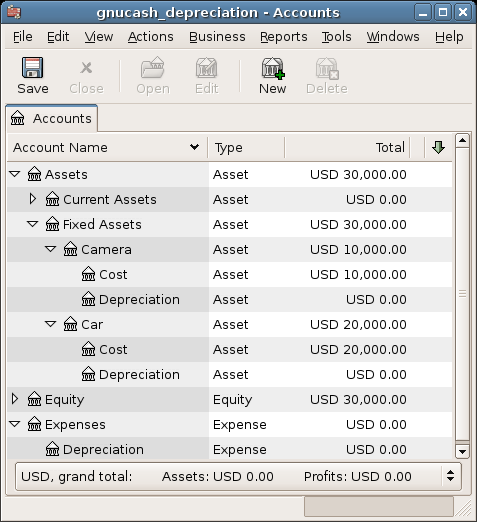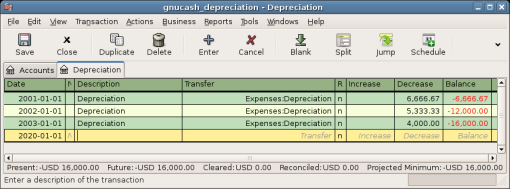## 16.4. Example

Let’s go ahead and step through an example. Imagine you are a photographer and you use a car and an expensive camera for your personal business. You will want to track the depreciation on these items, because you can probably deduct the depreciation from your business taxes.

The first step is to build the account hierarchy (as shown in the previous section, replace ITEM1 and ITEM2 with car and camera). Now, record the purchase of your assets by transferring the money from your bank account to the appropriate Asset Cost accounts for each item (eg: the Assets:Fixed Assets:Car:Cost account for the car). In this example, you start with \$30k in the bank, the car cost \$20k and the camera cost \$10k and were both purchased on January 1, 2000.

Figure 16.1. Asset Accounts Before DepreciationThe asset accounts still only have the investments stored.

Looking at the tax codes, we realize that we must report depreciation on these items using the sum of digits scheme, over a 5 year period. So, the yearly depreciation amounts for the car come to \$6667, \$5333, \$4000, \$2667, \$1333 for years 1 to 5 respectively, rounded to the nearest dollar. The yearly depreciation amounts for the camera are \$3333, \$2667, \$2000, \$1333, \$667. Consult the previous section on Depreciation Schemes for the formula for calculating these values.

For each accounting period (IE: fiscal year) you record the depreciation as an expense in the appropriate Accrued Depreciation account (eg: the Assets:Fixed Assets:Car:Depreciation account for the car). The two windows below show your car’s accrued depreciation account and the main window after the third year (IE: three periods) of depreciation using this sum of digits scheme.

Figure 16.2. Asset Depreciation In The RegisterThis account shows a list of depreciation transactions.

Figure 16.3. Accounts Tab After Asset DepreciationThe asset accounts got decreased by the depreciation.

NoteA Word of Caution: Since depreciation and tax issues are closely related, you may not always be free in choosing your preferred method. Fixing wrong calculations will cost a whole lot more time and trouble than getting the calculations right the first time, so if you plan to depreciate assets, it is wise to make sure of the schemes you will be permitted or required to use.